SHAREABLE DATA CENTER

## East Siberian Center for the Earth's Ionosphere Research (SDC ESCEIR)

Institute of Solar-Terrestial Physics SB RAS
Upper Atmosphere Physics and Radiowave Propagation Department

### Specific character ionosphere parameters measurements with the Irkutsk IS radar

In spite of the good potential possibilities, some of the station "Dnepr" design characteristics did not allow us to apply the usual special IS radar measurement methods. One of them is the design the station's antenna system, which excites and receives the linearly-polarized field. The Faraday effect causes the polarization vector to rotate, which leads to periodical signal fadings at the receiver input. Special purpose the IS radars usually measure two orthogonal polarizations to avoid this effect or they radiate and receive circularly polarized radiowaves. Thus for IS signal measurements at the "Dnepr" station, we have developed special methods for recording and processing IS signals in order to determine plasma ionospheric parameters.

 To solve this problem on the basis of the electromagnetic wave scattering statistical theory we have obtained the IS radar equation with Faraday effect. This equation gives us an opportunity to apply the IS method at radars with linear polarized antennas. The spectral density of the IS signal has the form: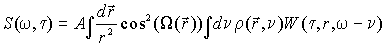(1) where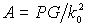, P -- radiation impulse power, G -- antenna gain, k0 -- wave vector,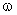-- frequency, c -- velocity of light;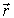-- radius-vector,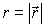,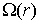-- polarization plane rotation angle of the wave,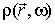-- spectral density of electron plasma fluctuations, W -- ambiguity function ,which has the form: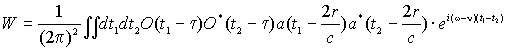(2)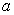-- complex envelope of the irradiated impulse,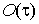-- Strobe function (window) of spectral processing,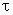-- delay of a given window from the beginning of the sounding impulse.
 The radar equation for the IS signal power with a given height is obtained by integration of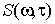over frequency. Integration takes into consideration that the scattering cross-section of a unit plasma volume has the form.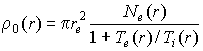(3) where re -- classical electron radius, and thatin these units is a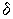-- function. Also, the IS signal power has the form.(4) where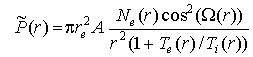.
 The desired ionospheric plasma parameters Ne, Te, Ti, and Vd, defining the fluctuation density, can be deduced from (1). When measuring, it is necessary that the sounding impulse spectrum be sufficiently narrower compared to. Because the spectrum widthwith the wavelength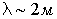is ~4-6KHz, the impulse length of ~1millisecond is chosen. The value of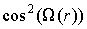in (1) is determined experimentally from additional measurements by solving (4) for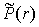. In these measurements we use a shorter impulse to obtain the necessary space resolution. On solving equation (4) (after deconvolution) equation (1) has the form.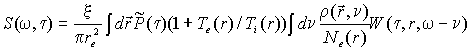(5) where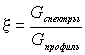is the ratio of two gains of two channels of measurements.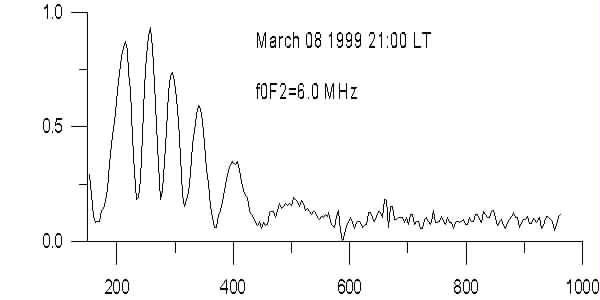Equations (4-5) from the basis for data post processing to obtain ionosphere parameters. On the one hand, the presence of one linear polarization gives rise to some complexity in algorithms for analyzing the IS signal by formulas (1-4); and, on the other, the determined character of polarization plane rotation gives us an opportunity to make absolute calibration of the electron density, while at the other radars one needs to use ionosonde data. In our case, the electron density is determined from equation (4), which gives a one-to-one correspondence between the polarization phase and electron density.

Composition | Objectives | Capabilities and samples | Request for work | Back to main page